# Test: Motion in a Straight Line - 3

## 30 Questions MCQ Test Physics Class 11 | Test: Motion in a Straight Line - 3

Description
Attempt Test: Motion in a Straight Line - 3 | 30 questions in 60 minutes | Mock test for NEET preparation | Free important questions MCQ to study Physics Class 11 for NEET Exam | Download free PDF with solutions
QUESTION: 1

### A particle covers half of the circle of radius r. Then the displacement and distance of the particle are respectively -

Solution:

When a particle travels a semicircle, the distance travelled is equal to the semi circumference of the circle while its displacement is equal to the distance between two diametric ends that is diameter 2r.

QUESTION: 2

### A hall has the dimensions 10m × 10m × 10 m. A fly starting at one corner ends up at a diagonally opposite corner. The magnitude of its displacement is nearly

Solution:

Given: Dimensions of Hall = 10 m × 10 m × 10 m
To find: Displacement from one corner to another corner
Hall is in the shape of Cube.
Length of longest diagonal of cube is given by,
Diagonal of cube=√3 × Length of edge
Displacement = √3 × 10
Displacement=10√3
So The answer B is correct

QUESTION: 3

### A car travels from A to B at a speed of 20 km h-1 and returns at a speed of 30 km h-1. The average speed of the car for the whole journey is

Solution:

Let distance from A to B be x

Time for travel from A to B= x/20

Time for travel from B to A= x/30

Hence,speed for total distance 2x is: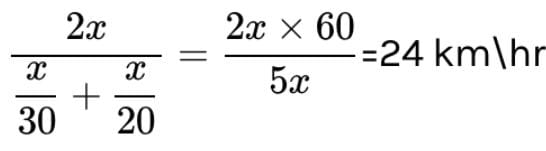QUESTION: 4

A car travels a distance of 2000 m. If the first half distance is covered at 40 km/hour and the second half at velocity v and if the average velocity is 48 km/hour, then the value of v is -

Solution:

Total distance covered is 2km, and first 1km is covered with speed of 40kmph thus total time taken is 1/40 hrs similarly as second half is travelled with velocity v time taken would be 1/v hrs. But we know that as average velocity is 48 kmph total time travelled can also be written as 2/48 hrs. Thus we get
1/40 + 1/v = 2/48
I.e 1/v = 1/24 - 1/40
= (40 - 24) / 40 x 24
Thus we get v = 40 x 24 / 16
= 60 kmph

QUESTION: 5

At an instant t , the co-ordinates of a particle are x = at2, y = bt2 and z = 0 , then its velocity at the instant t will be

Solution:

At some time instant t, the displacement of the particle from origin = √(at2 )2+ (bt2)2
I.e. s = t2√a2+b2
Thus we get v =  2t√a2+b2

QUESTION: 6

A car runs at constant speed on a circular track of radius 100 m taking 62.8 s on each lap. What is the average speed and average velocity on each complete lap?

Solution:

After each lap done by the car, displacement = 0 m
And distance covered = 2r = 2 x 3.14 x 100 m
Thus avg. velocity = 0 and avg. speed = 628 / 62.8 = 10m/s

QUESTION: 7

The displacement of a body is given by 2s = gt2 where g is a constant. The velocity of the body at any time t is

Solution:

Given that s = ½ gt2
Thus we get v = ds/dt = gt

QUESTION: 8

A particle is moving so that its displacement s is given as s = t3- 6t2 + 3t + 4 meter. Its velocity at the instant when its acceleration is zero will be-

Solution:

For the given particle, v = 3t2 - 12t + 3
And its acceleration, a = 6t - 12
Thus we get a = 0
At t = 2 sec,
Hence v at t = 2 sec
V = 3 x 4 - 12 x 2 + 3
= -9m/s

QUESTION: 9

A body starts from rest and is uniformly accelerated for 30 s. The distance travelled in the first 10s is x1, next 10 s is x2 and the last 10 s is x3. Then x1 : x2 : x3 is the same as

Solution:

Let's say that the uniform acceleration is a.
Now distance travelled in 0 - 10 sec, x1 = ½.a x 100 = 50a
Similarly, distance travelled in 0 - 20 sec,  = ½.a x 400 = 200a
Thus we get distance travelled in 10 - 20 sec, x2 = 200a - 50a = 150a
And, distance travelled in 0 - 30 sec,  = ½.a x 900 = 450a
Thus we get distance travelled in 20 - 30 sec, x3 = 450a - 200a = 250a
Hence we get the ratio as 1 : 3 : 5

QUESTION: 10

The displacement-time graph of a moving particle is shown below. The instantaneous velocity of the particle is negative at the point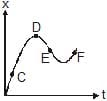Solution:

If we will draw a tangent of the point then ot will make angle x with t. Since only the shortest angle will be taken, thus it will also make 180-x.Since we now know that the slope= tan x, thus the slope at E = tan(180-x) =-tanx, thus the instantaneous velocity at E is negative

In the given question C and F have a positive slope so instantaneous velocity is positive. Since point  D has zero slope so instantaneous velocity is zero but E has negative so instantaneous velocity is negative at E.

QUESTION: 11

The variation of velocity of a particle moving along straight line is shown in the figure. The distance travelled by the particle in 4 s is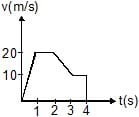Solution:

The distance traveled is equal to the area under the curve which is equal to
S = ½ x 20 x1 + 20 x 1 +½ x 10 x1 + 10 x 2
= 10 + 20 + 5 + 20
= 55m

QUESTION: 12

The displacement time graphs of two particles A and B are straight lines making angles of respectively 30º and 60º with the time axis. If the velocity of A is vA and that of B is vB then the value of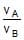is

Solution:

For some point of time say time t, the velocities of A and B would be va  and vb respectively.
Hence we see that for both the graphs we get va/t = tan 30 and vb/t = tan 60
Thus we get the required ratio as tan 30 / tan 60 = ⅓

QUESTION: 13

The v-t graph of a linear motion is shown in adjoining figure. The distance from origin after 8 seconds is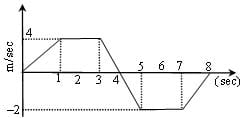Solution:

Distance = area under curve

=1/2(2+4)×4 + 1/2(2+4)×2

=18m

QUESTION: 14

The adjoining curve represents the velocity-time graph of a particle, its acceleration values along OA, AB and BC in metre/sec2 are respectively-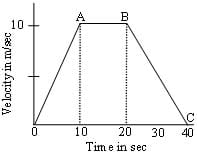Solution:

On a velocity-time graph the slope of the curve represents the acceleration. For OA the slope is 1, while for AB its 0 and for BC its ½.

QUESTION: 15

In the following velocity-time graph of a body, the distance and displacement travelled by the body in 5 second in meters will be -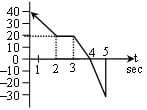Solution:

From the given velocity graph we get that displacement is w=equal to the area under the curve taken with sign while distance is area under the curve after the modulus is taken at first.
Thus displacement is
( ½ x 20 x 2 + 20 x 3 + ½ x 20 x 1) - (½ x 30 x 1) = 90 - 15 = 75m
And the distance will be
( ½ x 20 x 2 + 20 x 3 + ½ x 20 x 1) + (½ x 30 x 1) = 90 + 15 = 105m

QUESTION: 16

If position time graph of a particle is sine curve as shown, what will be its velocity-time graph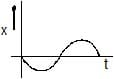Solution:

We know position time graph is sine graph and velocity is exact differential of position wrt time i.e. differentiation of sine which is negative of cosine. Hence the velocity time graph will be a negative cosine graph.

QUESTION: 17

A particle, after starting from rest , experiences, constant acceleration for 20 seconds. If it covers a distance of S1, in first 10 seconds and distance S2 in next 10 sec, then

Solution:

Let constant acceleration is a

distance travels in 20 seconds

s = 0*20+a*20²/2

s = 200a -------------(1)

distance travels in first 10 seconds

s1 = 0*10+a*10²/2

s1 = 50a -----------------(2)

distance travels in next 10 seconds

s2 = s-s1

s2 = 200a-50a = 150a ----------------(3)

s2/s1 = 150a/50a = 3

QUESTION: 18

A body sliding on a smooth inclined plane requires 4sec to reach the bottom after starting from rest at the top. How much time does it take to cover one fourth the distance starting from the top

Solution:

As we know initial velocity is zero hence total distance covered, s = ½ g.t
I.e s depends upon the t
Thus when distance is decreased to ¼ , time decreases to ½ .
I.e 2 sec.

QUESTION: 19

The initial velocity of a particle is 10 m/sec and its retardation is 2 m/sec2. The distance covered in the fifth second of the motion will be

Solution:

Speed after 4 sec, v = 10 - 2 x 4
= 2m/s
Thus distance traveled in fifth second, s = 2 x 1 - ½ x 2 x 1
= 2 - 1
= 1m

QUESTION: 20

A body starts from rest, the ratio of distances travelled by the body during 3rd and 4thseconds is :

Solution:

The velocity after 2 sec = 2a and velocity after 3 sec = 3a
For some constant acceleration a,
Now distance travelled in third second, s3 = 2a + ½ a
= 5/2 a
Similarly distance travelled in fourth second, s4= 3a + ½ a
= 7/2 a
Hence the reqiuired ratio is 5/7

QUESTION: 21

A body is dropped from a height h under acceleration due to gravity g. If t1 and t2 are time intervals for its fall for first half and the second half distance, the relation between them is

Solution:

h=1/2g(t1+t2)2

2h/g=(t1+t2)2------(1)

h/2=1/2gt12

t12=h/g---------(2)

From 1st and 2nd

t12=(t1+t2)2/2

t1=(t1+t2)/1.41

1.41t1=t1+t2

t1=t2/.41

So

t1=2.414t2

QUESTION: 22

Two bodies of different masses ma and mb are dropped from two different heights, viz a and b. The ratio of times taken by the two to drop through these distances is

Solution:

Time taken by anybody to fall from a height h is
T = 2gh which is independent of the mass
Hence the required ratio will be that of option c

QUESTION: 23

A body is thrown upward and reaches its maximum height. At that position-

Solution:

Acceleration due to gravity takes place every moment on earth whether you are throwing yourself up or else just diving into the pool. It is also indeed true that acceleration causes a change in velocity. But it happens that the change from a negative vector of velocity to positive one undergoes a period when the object/body literally has a Zero velocity for a very very short amount of time.

QUESTION: 24

Two trains each of length 50 m are approaching each other on parallel rails. Their velocities are 10 m/sec and 15 m/sec. They will cross each other in -

Solution:

Total distance =50+50=100
Total speed=10+15=25 s.
As we know, s= d/t [s = speed (meters/second)
d = distance traveled (meters)
t = time (seconds)]
we get t= d÷s
so, t=100/25
t= 4 sec

QUESTION: 25

A car A is going north-east at 80 km/hr and another car B is going south-east at 60 km/hr. Then the direction of the velocity of A relative to B makes with the north an angle a such that tan a is -

Solution:

Both the cars are moving in perpendicular directions and thus we stop b and see speed of a wrt b, we get the direction of a is 80.cos 45 + 60.cos 45 east and 80.sin 45 - 60.sin 45.
That is the directions are 140.cos 45 east and 20.sin 45 north.
Thus the angle with north is tan-1 20/140
= tan-1 1/7

QUESTION: 26

An object A is moving with 10 m/s and B is moving with 5 m/s in the same direction of positive x-axis. A is 100 m behind B as shown. Find time taken by A to Meet B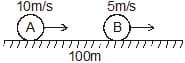Solution:

vA​=10m/s
vB​=5m/s
Here, Vrelative=10m/s-5m/s
=5m/s
Now,t=S/V=100m/5m/s=20sec

QUESTION: 27

A ball is thrown upwards. It returns to ground describing a parabolic path. Which of the following remains constant ?

Solution:

Only the horizontal component remains constant as as there is no component of acc. in horizontal direction. The g aaceleration due to gravity acts down words hence there is no accln in horizontal direction, the velocity changes in vertical direction as goes up and down while in horizontal it goes only forward.

QUESTION: 28

The angle of projection of a body is 15º . The other angle for which the range is the same as the first one is equal to-

Solution:

We know that horizontal range R depends upon sin 2a where a is angle of projection. Also we know that sin 2a repeats its value for all π/2 -a. thus as here a = 15, the value of range will be equal to the same for 90 -15 = 75.

QUESTION: 29

A ball is thrown at an angle of 45º with the horizontal with kinetic energy E. The kinetic energy at the highest point during the flight is-

Solution: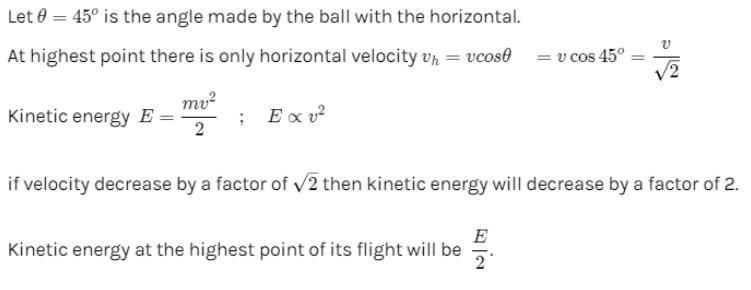QUESTION: 30

The maximum range of a projectile is 22 m. When it is thrown at an angle of 15º with the horizontal, its range will be-

Solution:

In the projectile motion the horizontal range of the object is determined as
R = u2.sin2x / g
Also we know that range is maximum when angle is 45°
Hence we get R = 22m =  u2.sin 90° / g
We get  u2 / g = 22
Now when the angle is 15°
We get R = u2.sin 30° / g
= u2/ 2g
= 11mUse Code STAYHOME200 and get INR 200 additional OFF Use Coupon Code